# Physics - Electromagnetism - Applications of charged particle motion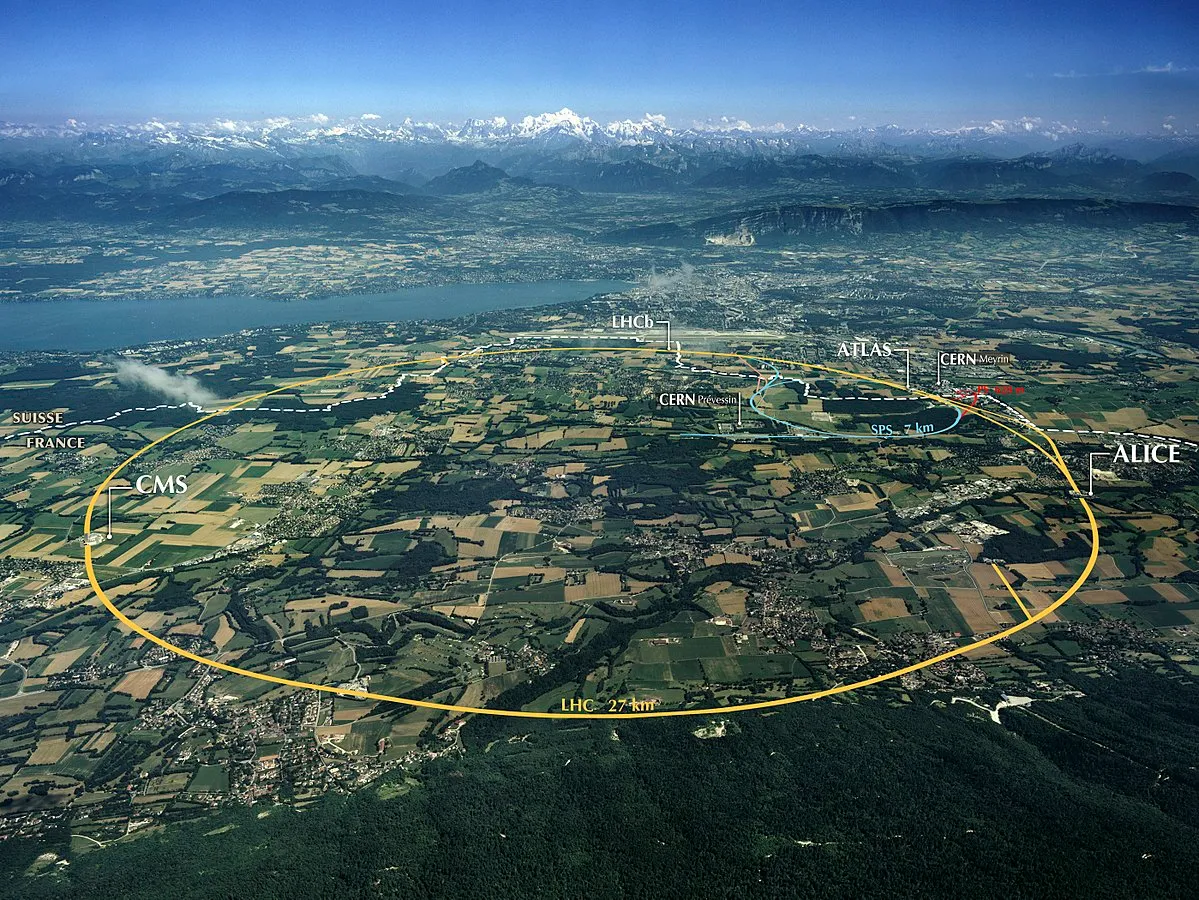Image source:## Introduction

Hello it's a me again Drifter Programming! Today we continue with Electromagnetism to get into Applications of charged particle motion. Last time we covered the actual motion of such particles and so I highly suggest you to read the previous post before this one. The main focus of today's post will be CERN. So, without further do, let's get straight into it!

## CERN

I found out about CERN when I was in Highschool. A lot of Greek Scientists leave Greece to go to Research Facilities like CERN, the NASA etc., cause here in Greece the choices are very limited. Some Students around the Highschools in my region had sent Students to watch a presentation about CERN and to also make a video call with a Greek scientist that does research there. Being very passioned about Physics it was me that went from my Class to the presentation!

So, what is CERN? CERN is a European Organization for Nuclear Research. Physicists and engineers do experiments on fundamental particles using large powerful particle accelerators to seek answers about the fundamental structure of the universe. Using complex scientific instruments particles are made to collide together at nearly the speed of light. This process gives the physicists information about how particles interact and so gives them an insight into the fundamental laws of nature. The main scientific instruments used at CERN are particle accelerators and detectors.

CERN is at the borders of France and Switzerland near Geneva and I would love to go there some day! To learn more about CERN I would suggest you to go and watch the 4-minute video at the "about" page:

CERN in general has a very cool website that explains everything!

## Cyclotrons and Synchrotrons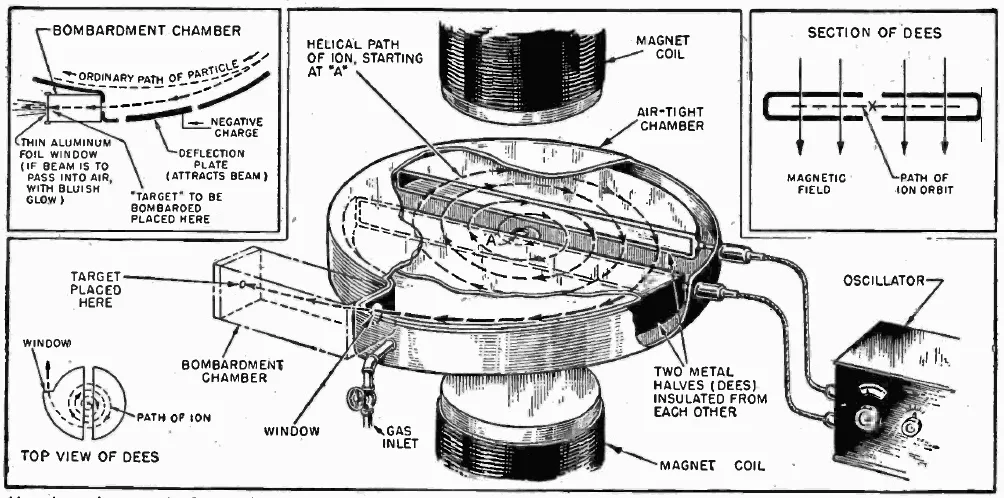Image source:So, how do these accelerators at CERN work? The simplest form of particle accelerators are the Cyclotrons that are D-shaped chambers (or dees) with a strong vertical magnetic field. The protons get injected into the center and are accelerated by the voltage between the dees. By moving perpendicular to the magnetic field (vertical field) they follow a semicircular path due to the magnetic field. The small gap in-between of those dees causes the particles to accelerate again, because of high voltage. The magnetic field is static, whilst the electric field has a rapidly varying frequency. As we said last time the radius of the circular motion depends on the velocity of the particles cause they don't lose or gain energy and also don't lose mass. The actual kinetic energy gain/increase is due to the electric field in the gab between the electrodes. The protons/particles travel faster and faster each time they pass this gap and so the radius around the dees increases until they are ejected out as a high speed proton beam. Such cyclotrons are a good sourec of high-energy beams for nuclear physics experiments and for example used to make short-lived radioisotopes for medical imaging and research.

An improvement upon the cyclotron is the so called synchrotron. The guiding/bending of the magnetic is now time-dependent. This means that the particles are bended into a closed path and synchronized to a particle beam of increasing kinetic energy. The synchrotron is a concept that enables us to construct large-scale facilities cause the bending, beam focusing and acceleration can now be separated into different components.

## Cavity Magnetron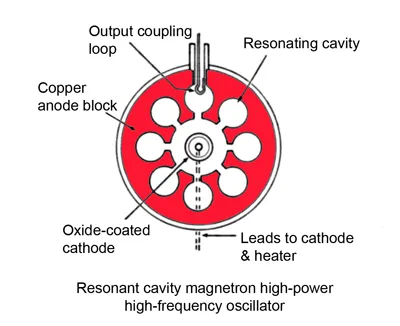Image source:A cavity magnetron is a high-powered vacuum tube. It generates microwaves using the interaction of a stream of electrons with a magnetic field. The cavity magnetrons consist of a hot cathode with a high negative potential created by a high-voltage, direct-current power supply. The cathode is built into the center of an evacuated, lobed, cicular chamber. A permanent magnet imposes a parallel magnetic field that causes the electrons to spiral outward in a circular path, because they are attracted by the positive outer part of the chamber. Spaced around the rim of the chamber are cylindrical cavities, which are open along their length and connect the common cavity space. As electrons sweep past these openings the form into groups. The actual sizes of those cavities determine the resonant frequency and so the frequency of the emitted microwaves. The only thing that such a magnetron needs is a power supply, cause it's a self-oscillating device. We use magnetrons in a lot of practical applications like radars, heating and lighting.

## Mass Spectrometry

To measure the mass-to-charge ratio of charged particles we use an analytical technique called Mass spectrometry. It is used to determine the masses of particles and thus to determine the elemental composition of a smale or molecule. Mass analyzers separate the ions according to their mass-to-charge ratio. The motion of those charged particles inside of electric and magnetic fields depends on Newton's 2nd law and the Lorentz which are given by the equations: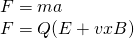Equating the above expressions we get:The term m/Q determines the motion of the charged particles. There are many types of mass analyzers that are using static or dynamic, electric or magnetic fields, but all of them operate according to the above equation.

A schematic of a IRMS (isotope ratio mass spectrometer) is as following:Image source:Suppose we want to calculate the m/q ratio of isotopic carbon dioxide. Each molecule has the same charge, but different masses. The mass spectrometer allows us to measure the mass-to-charge ratio of each particle. Since the charge is known we can measure the absolute mass. By counting the number of particles of each mass we can that way calculate the total mass of this isotope.

## Magnetic lens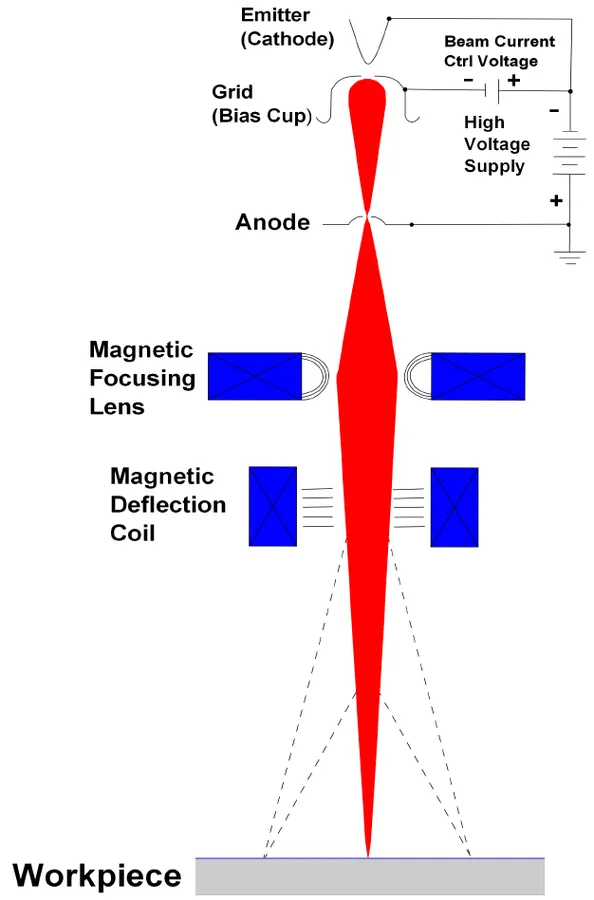Image source:We covered electrostatic lenses and explained how the cathode ray tubes of "older" TV's work. Using lenses in general we focus light and electrons. We want to keep the same energy, but change the initial angles so that they come together in a small spot. A magnetic lens is build of a cylindrically symmetric electromagnet with very sharp circular pole tips which produce a strong, nonuniform field in a small region. Electrons which travel vertically through this region are focused. Suppose that two electrons are being emmited in different angles. As they reach the beginning of the field they get deflected by the horizontal component of the field so that they get impulsed towards the axis. The two divergent electorns are brought into parallel paths and so this action is like a lens with an object at the focal point.

## REFERENCES:

Mathematical equations that I had in this post where drawn using quicklatex!

### Electric fields:

Getting into Electromagnetism -> electromagnetim, electric charge, conductors, insulators, quantization

Coulomb's law with examples -> Coulomb's law, superposition principle, Coulomb constant, how to solve problems, examples

Electric fields and field lines -> Electric fields, Solving problems around Electric fields and field lines

Electric dipoles -> Electric dipole, torque, potential and field

Electric charge and field Exercises -> examples in electric charges and fields

### Electric flux:

Electric flux and Gauss's law -> Electric flux, Gauss's law

Applications of Gauss's law (part 1) -> applying Gauss's law, Gauss applications

Applications of Gauss's law (part 2) -> more Gauss applications

Electric flux exercises -> examples in electric flux and Gauss's law

### Electric potential:

Electric potential energy -> explanation of work-energy, electric potential energy

Calculating electric potentials -> more stuff about potential energy, potential, calculating potentials

Millikan's Oil Drop Experiment -> Millikan's experiment, electronvolt

Cathode ray tubes explained using electric potential -> cathode ray tube explanation

Electric potential exercises (part 1) -> applications of potential

Electric potential exercises (part 2) -> applications of potential gradient, advanced examples

### Capacitance:

Capacitors (Condensers) and Capacitance -> Capacitors, capacitance, calculating capacitance

How to solve problems around Capacitors -> combination, solving problems, simple example

Electric field energy and density -> Electric field energy, energy density

Dielectric materials -> Dielectrics, dielectric constant, permittivity and strength, how to solve problems

Electric capacitance exercises -> examples in capacitance, energy density and dielectrics

### Current, resistance and EMF:

Electric current -> Electric current, current density

Electrical resistivity and conductivity -> Electrical resistivity, conductivity, thermal coefficient of resistivity, hyperconductivity

Electric resistance -> Resistance, temperature, resistors

Electromotive Force (EMF) and Internal resistance -> Electromotive force, internal resistance

Power and Wattage of Electronic Circuits -> Power in general, power/wattage of electronic circuits

Electric current, resistance and emf exercises -> exampes in all those topics

### Direct current (DC) circuits:

Resistor Combinations -> Resistor combinations, how to solve problems

Kirchhoff's laws with applications -> Kirchhoff's laws, how to solve problems, applications

Electrical measuring instruments -> what are they?, types list, getting into some of them, an application

Electronic circuits with resistors and capacitors (R-C) -> R-C Circuit, charging, time constant, discharging, how to apply

RC circuit exercises -> examples in Kirchhoff, charging, discharging capacitor with/without internal resistance

### Magnetic field and forces:

Magnetic fields -> Magnetism, Magnetic field

Magnetic field lines and Gauss's law of Magnetism -> magnetic field lines, mono- and dipoles, Flux, Gauss's law of magnetism

The motion of charged particles inside of a magnetic field -> straight-line, spiral and helical particle motion

And this is actually it for today's post!

Next time we will get into the Magnetic force applied to a conductor that has electric current.

Bye bye!

H2
H3
H4
3 columns
2 columns
1 column